# 978-0123869449 Chapter 5 Part 4

Document Type
Homework Help
Book Title
Authors
Michael F. Modest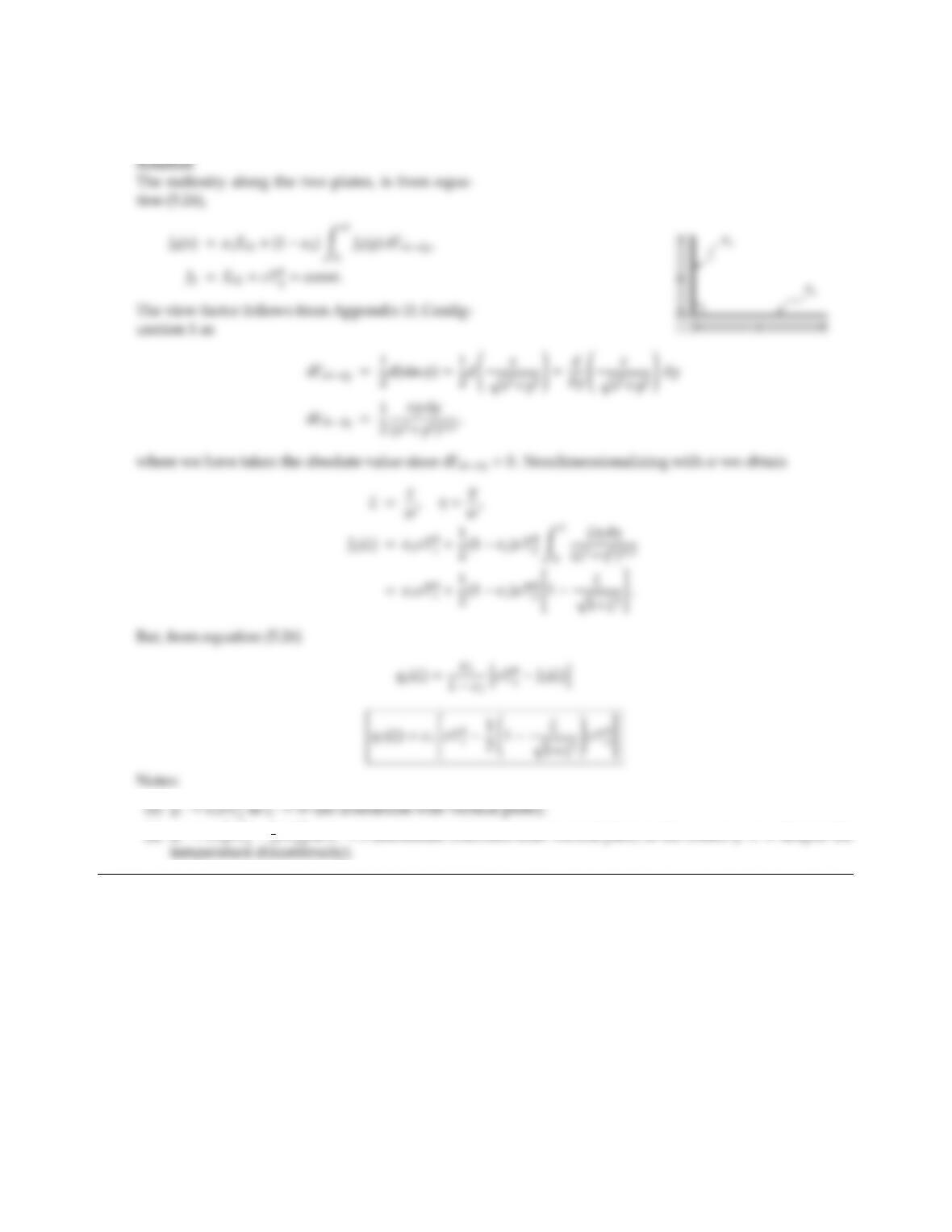5.33 Consider Configuration 33 in Appendix D with h=w. The bottom wall is at constant temperature T1and has
emittance ǫ1; the side wall is at T2=const and ǫ2. Find the exact expression for q1(x) if ǫ2=1.
(2) q1ǫ1σT4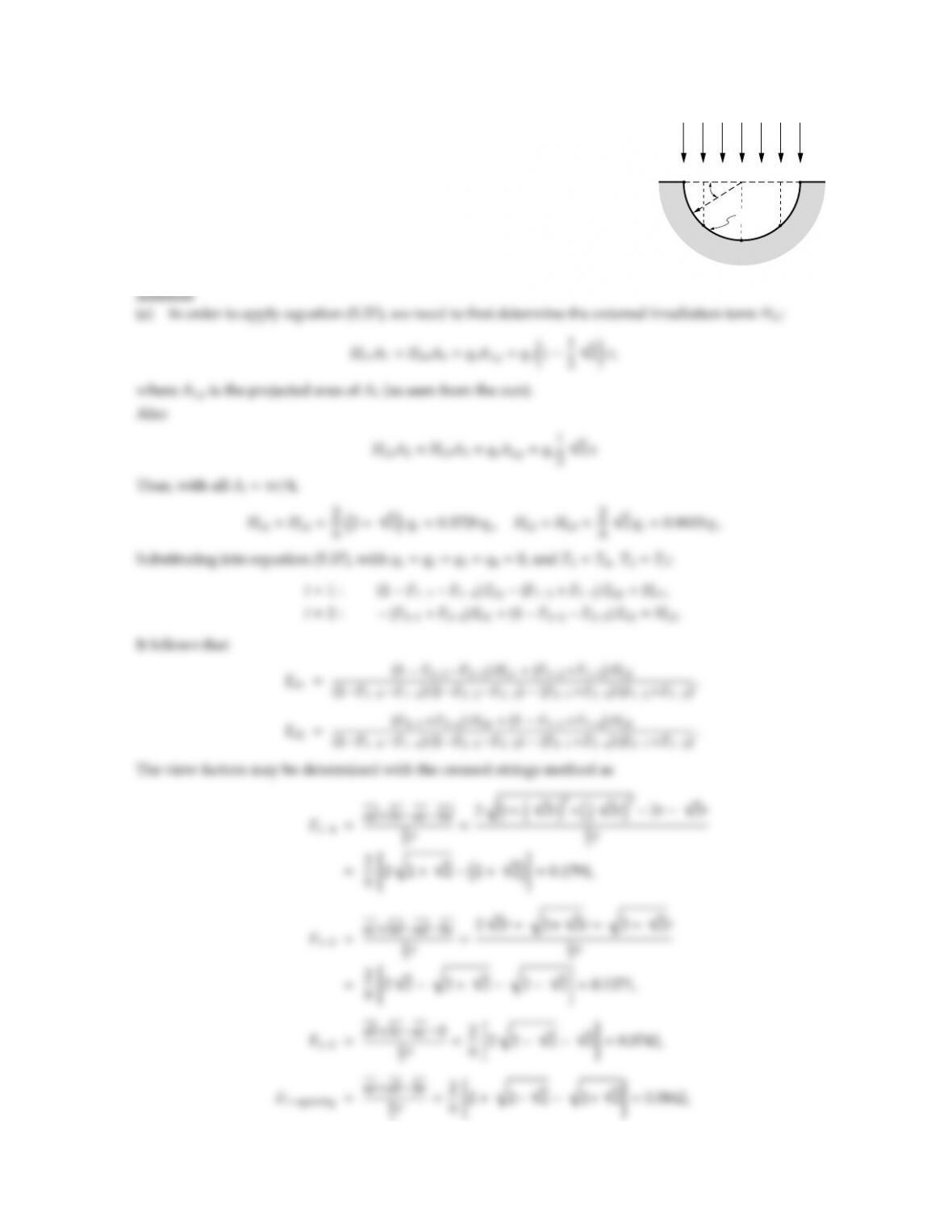CHAPTER 5 155
5.34 An infinitely long half-cylinder is irradiated by the sun as shown in the figure,
with qsun =1000 W/m2. The inside of the cylinder is gray and diuse, the
outside is insulated. There is no radiation from the background. What is the
equilibrium temperature distribution along the cylinder periphery,
(a) using four isothermal zones of 45each,
(b) using the exact relations. (Hint: Use dierentiation as in the kernel
approximation method).
qsun
ϕ
r
, T( )
ϕ
a
b
c
d
e
A1
A2A3
A4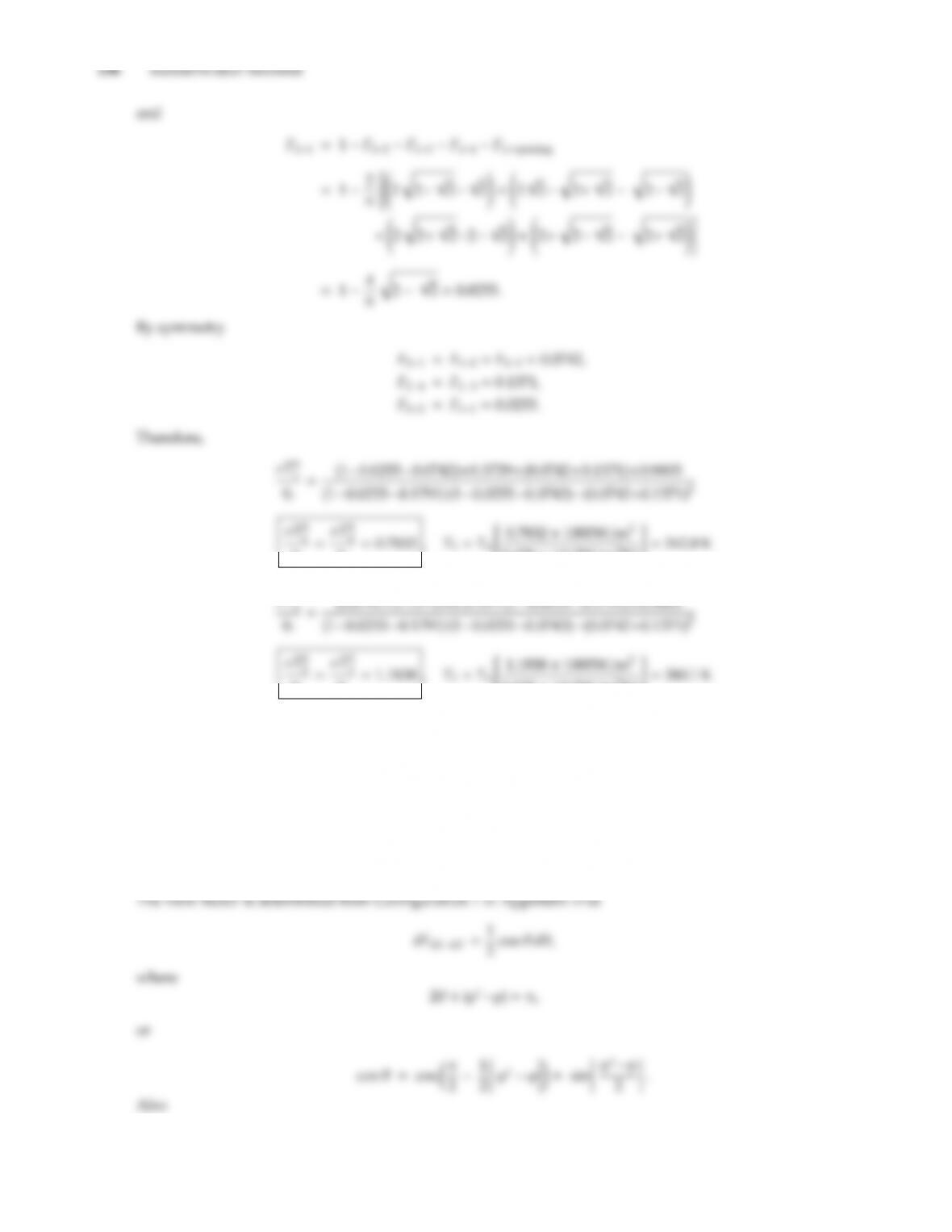qs
qs
5.670 ×108W/m2K4#=342.8 K
σT4
qs
qs
5.670 ×108W/m2K4#=380.1 K
(b) From equation (5.21) it follows with
Ho(ϕ)=qsdA sin ϕ,
H(ϕ)dA =qssin ϕdA +ZA
or
H(ϕ)=qssin ϕ+ZA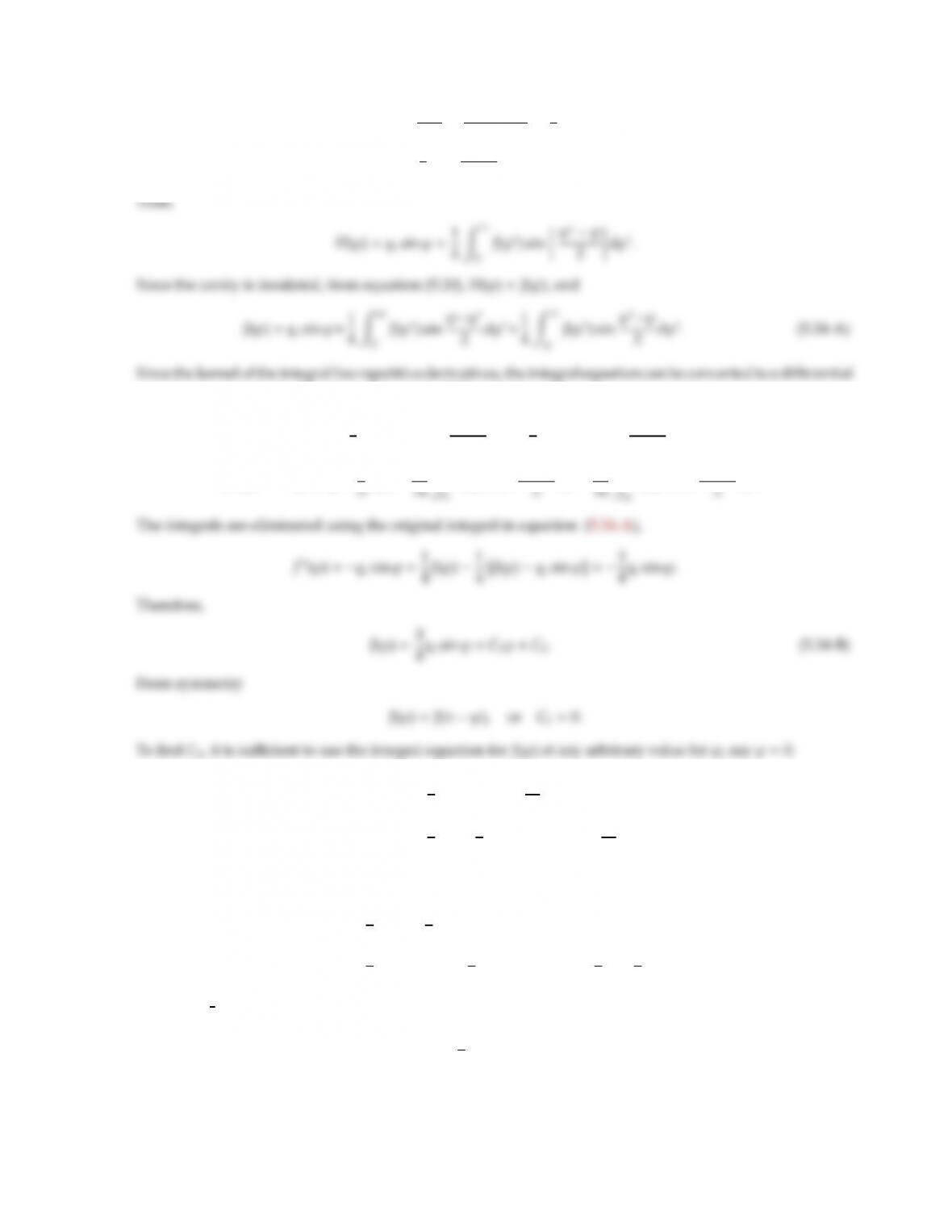CHAPTER 5 157
dθ=dA
p
S=rdϕcos θ
2rcos θ=1
2dϕ
4sin
ϕϕ
2
dϕ.
equation (as done in the kernel approximation). Dierentiating twice
J(ϕ)=qscos ϕ+1
8Zϕ
0
J(ϕ) cos ϕϕ
2dϕ1
8Zπ
ϕ
J(ϕ) cos ϕϕ
2dϕ,
J′′(ϕ)=qssin ϕ+1
J(ϕ) sin ϕϕ
J(ϕ) sin ϕϕ
J(0) =C2=1
4Zπ
0
J(ϕ) sin ϕ
2dϕ
=1
4Zπ
03
4qssin ϕ+C2sin ϕ
2dϕ.
Setting ϕ=2η, with sin ϕ=2 sin ηcos η,
C2=1
2Zπ/2
03
2qssin ηcos η+C2sin ηdη
=1
4qssin3η
π/2
0+1
2C2cos η
π/2
0=1
4qs+1
2C2.
Thus C2=1
2qs, and
J(ϕ)=1
4qs(2 +3 sin ϕ).
[However, to avoid errors it is generally preferable to substitute equation (5.34-B) into equation (5.34-A) for
arbitrary ϕ: if the constants C1(=0) and C2are determined without contradictions, one is almost certain that noerrors were made!] For an insulated wall, radiosity is equal to blackbody emissive power [cf. equation (5.31)],
and
σT4(ϕ)=J(ϕ)=1
4qs(2 +3 sin ϕ)
In this particular example, the exact solution is actually simpler than the net radiation approach. This is, of
course, not generally the case. Evaluating the exact solution for ϕ=π/8 and 3π/8 [center of the nodes in part
(a)], we obtain for comparison
σT4
qsπ
8=σT4
qs7π
8=0.7870,
σT4
qs3π
8=σT4
qs5π
8=1.1929,
which is in excellent agreement with the approximate solution.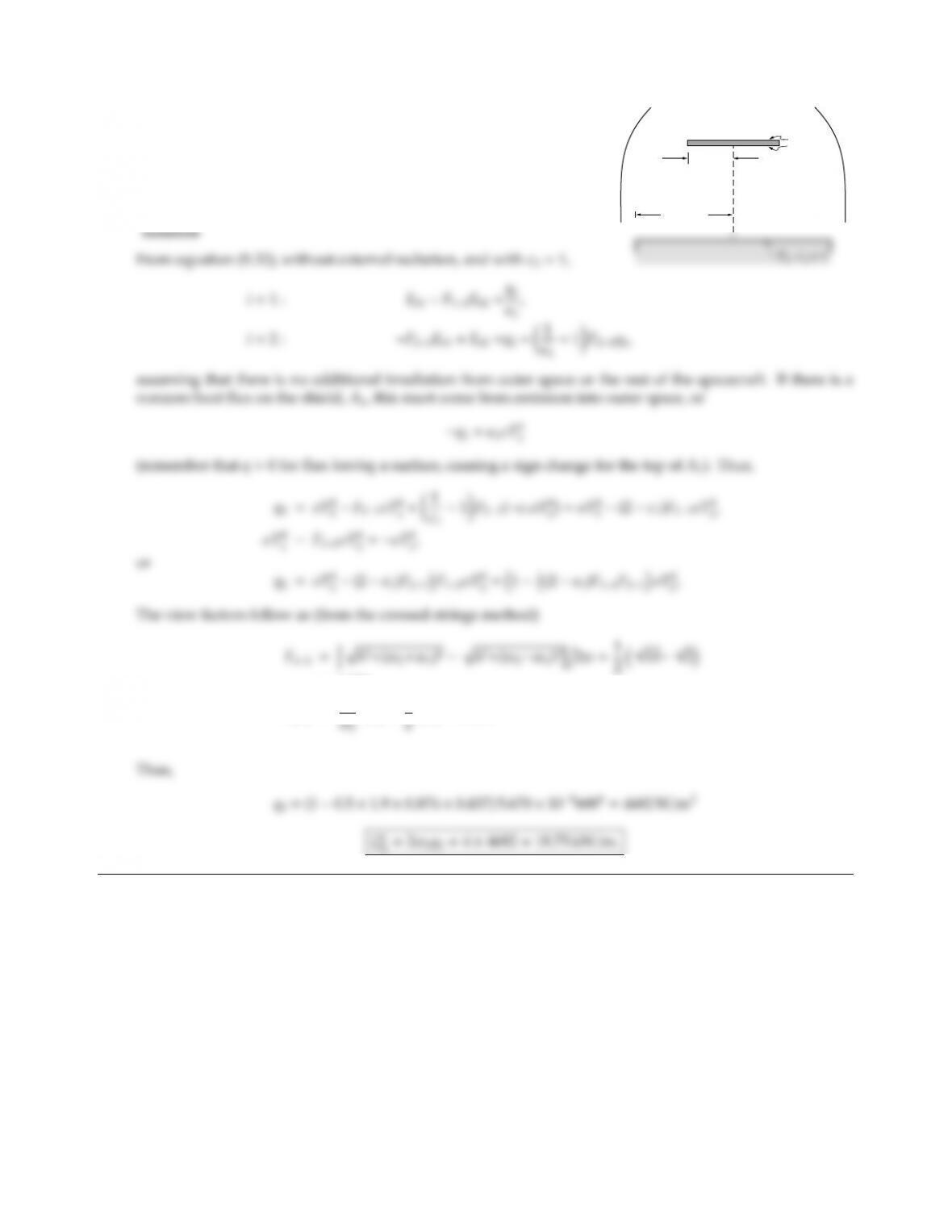CHAPTER 5 159
5.35
w1 =1 m
h = 1 m
w2 = 2 m
0 K
1 = 0.1
0 K
To calculate the net heat loss from a part of a spacecraft, this part may
be approximated by an infinitely long black plate at temperature
T2=600 K, as shown. Parallel to this plate is another (infinitely
long) thin plate that is gray and emits/reflects diusely with the
same emittance ǫ1on both sides. You may assume the surroundings
to be black at 0 K. Calculate the net heat loss from the black plate.
=0.874,
F21=w1
F12=1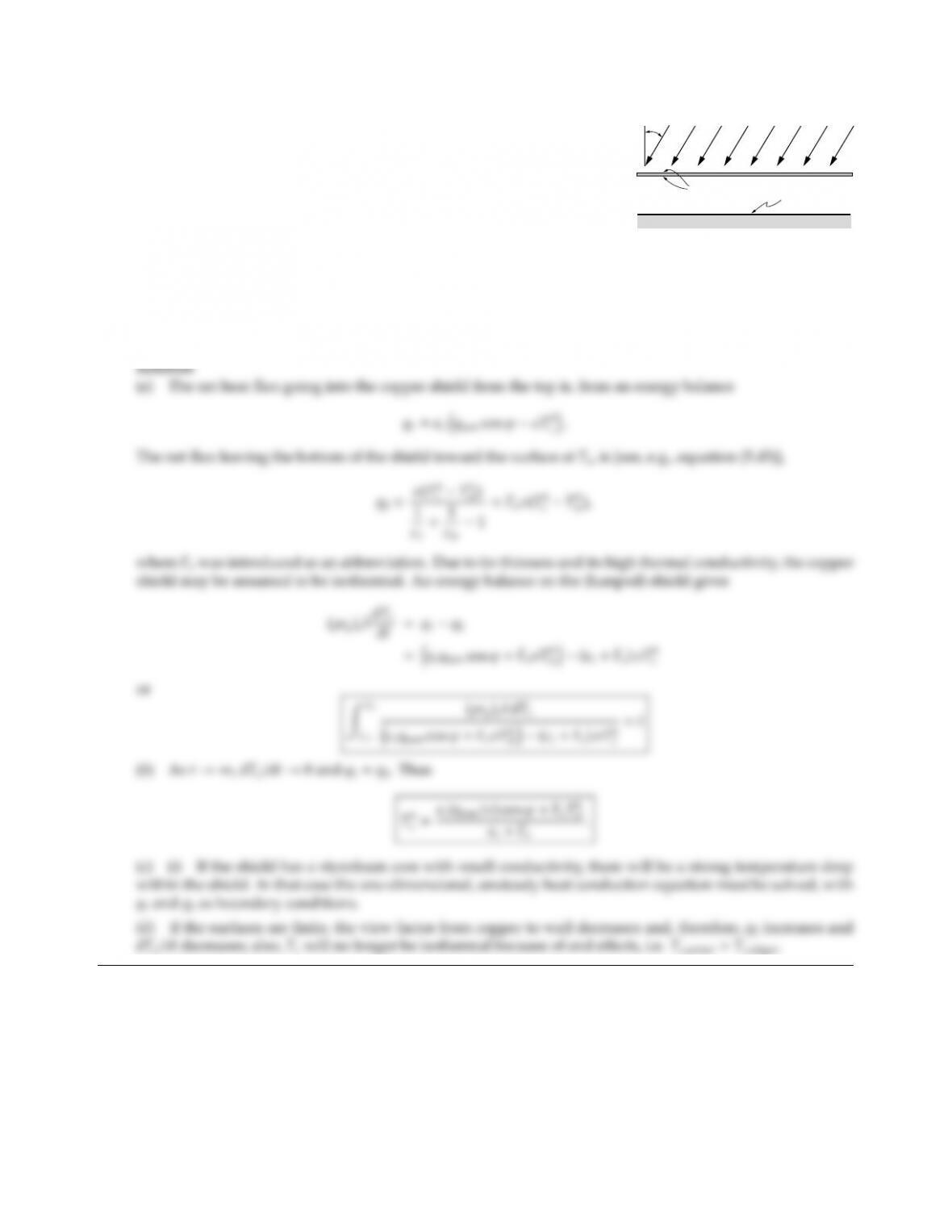5.36
Tc, c
qsun
ϕ
Tw , w
A large isothermal surface (exposed to vacuum, temperature Tw,
diuse-gray emittance ǫw) is irradiated by the sun. To reduce the
heat gain/loss from the surface, a thin copper shield (emittance ǫc
and initially at temperature Tc0) is placed between surface and sun as
shown in the figure.
(a) Determine the relationship between Tcand time t(it is sucient
to leave the answer in implicit form with an unsolved integral).
(b) Give the steady state temperature for Tc(i.e., for t→ ∞).
(c) Briefly discuss qualitatively the following eects:
(i) The shield is replaced by a moderately thick slab of styrofoam coated on both sides with a very thin
layer of copper.
(ii) The surfaces are finite in size.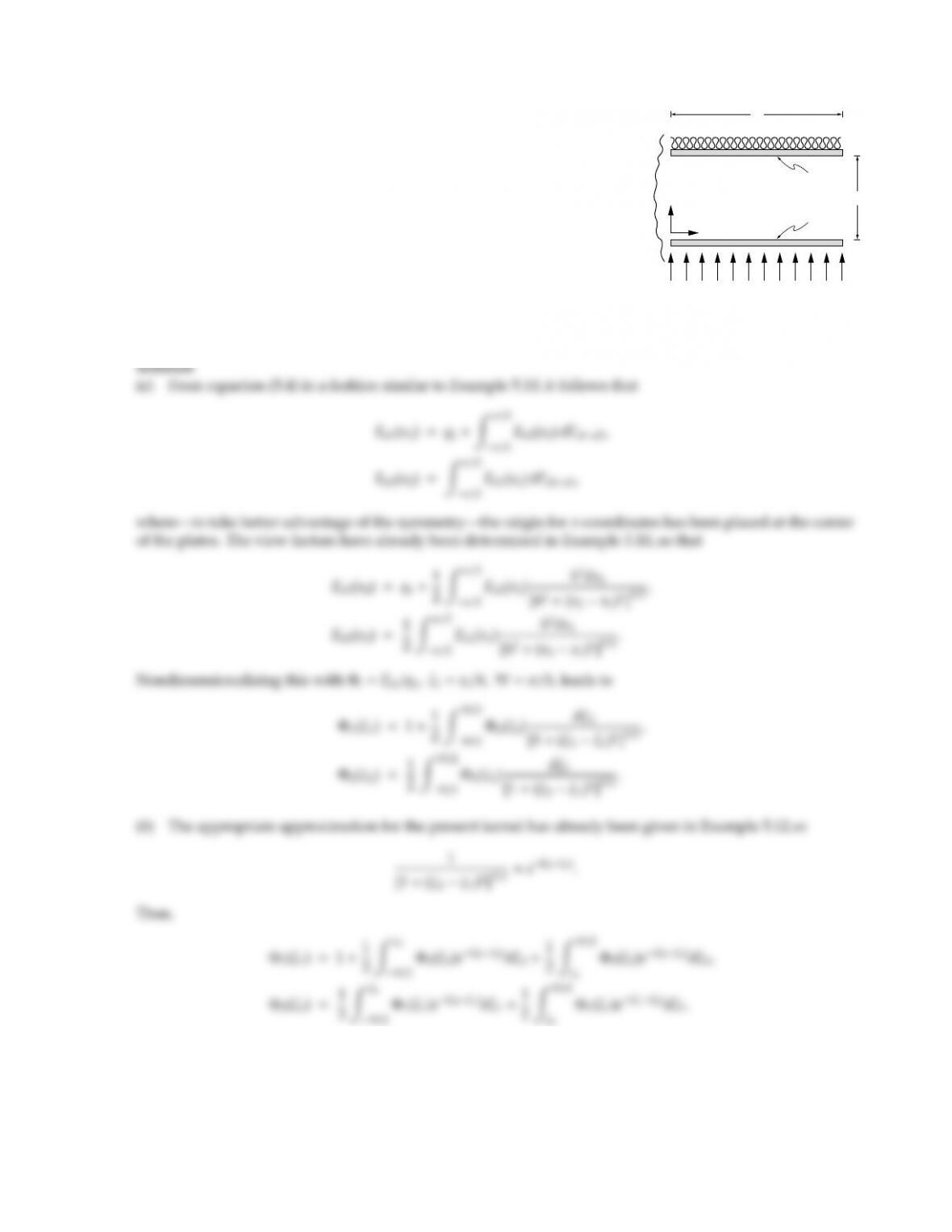CHAPTER 5 161
5.37
q1
Insulation
y
x
L
h
A2, 2
A1, 1
0 K
Consider two infinitely long, parallel, black plates of width Las shown.
The bottom plate is uniformly heated electrically with a heat flux of
q1=const, while the top plate is insulated. The entire configuration is
placed into a large cold environment.
(a) Determine the governing equations for the temperature variation
across the plates.
(b) Find the solution by the kernel substitution method. To avoid
tedious algebra, you may leave the final result in terms of two
constants to be determined, as long as you outline carefully how
these constants may be found.
(c) If the plates are gray and diuse with emittances ǫ1and ǫ2, how can the temperature distribution be
determined, using the solution from part (b)?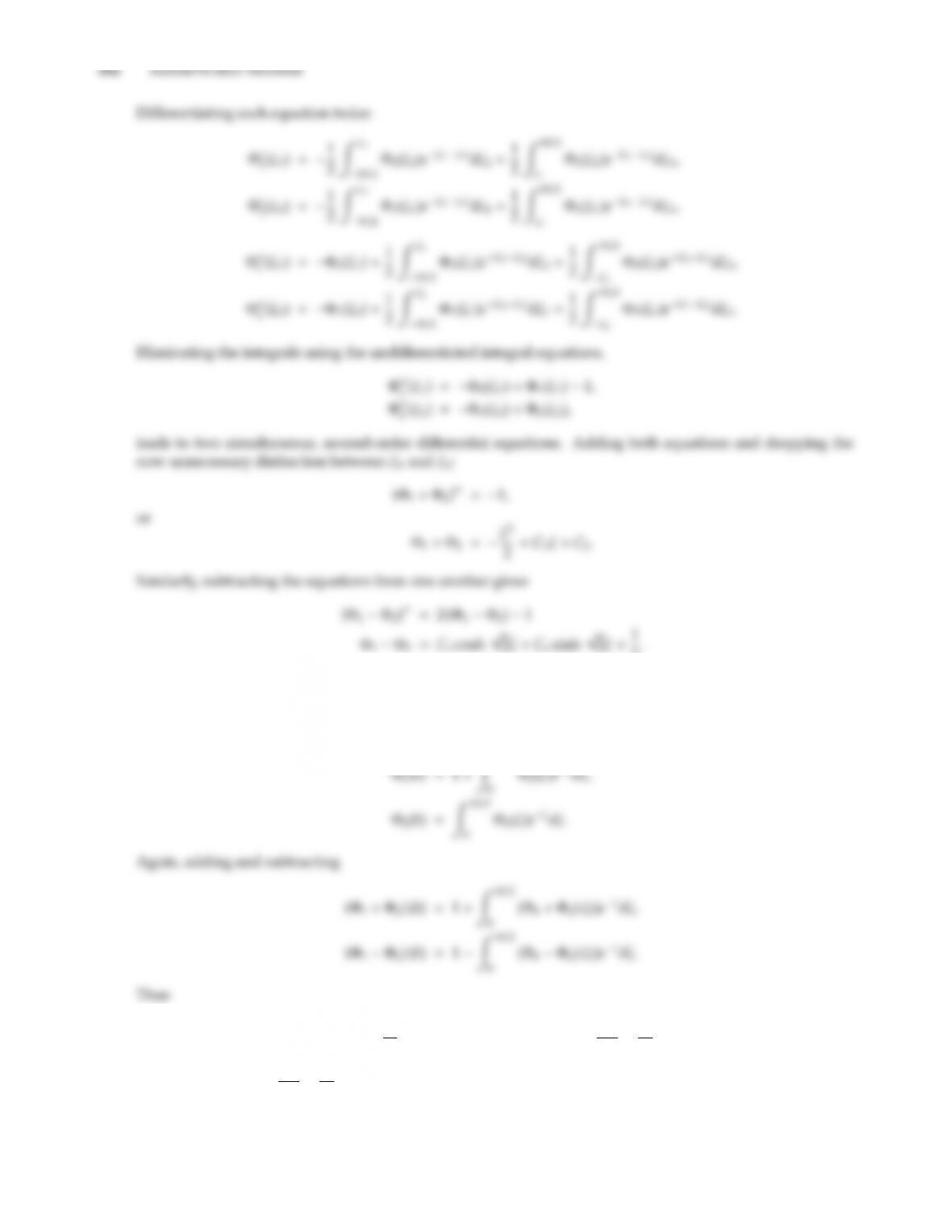2.
Because of symmetry Φi(ξ)= Φi(ξ), which requires that C1=C4=0. The other two constants must be found
by substitution into the undierentiated integral equation at any value for ξ. Choosing ξ=0 and using the
symmetry condition [which shows that R0
W/2( )dξ=RW/2
C2=1+ZW/2
0 C2ξ2
2!eξdξ=1+C21eW/2+ W2
8+W
2+1!eW/21,
C2=W2
8+W
2+1.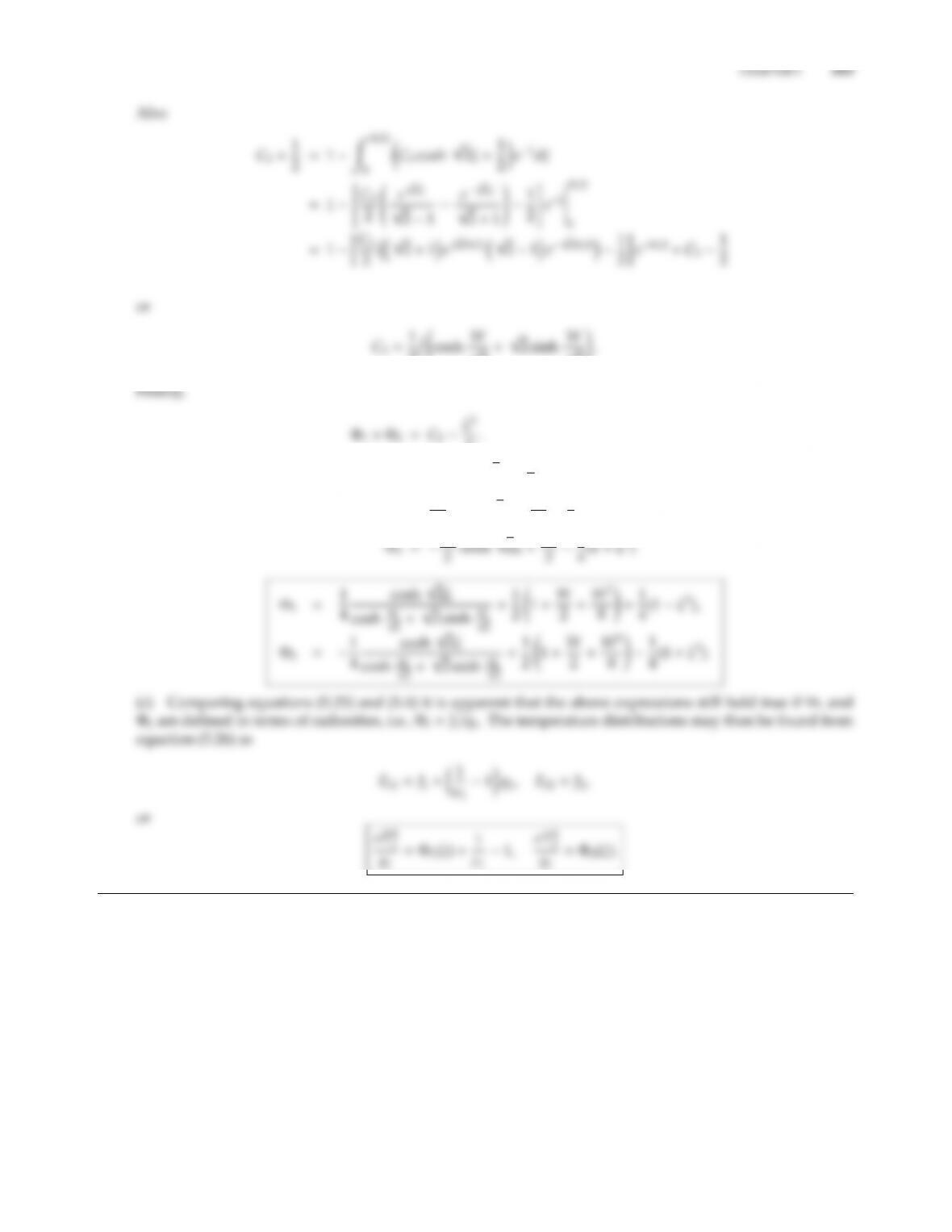2. cosh W
2.
Φ1Φ2=C3cosh 2ξ+1
2,
Φ1=C3
2cosh 2ξ+C2
2+1
4(1 ξ2)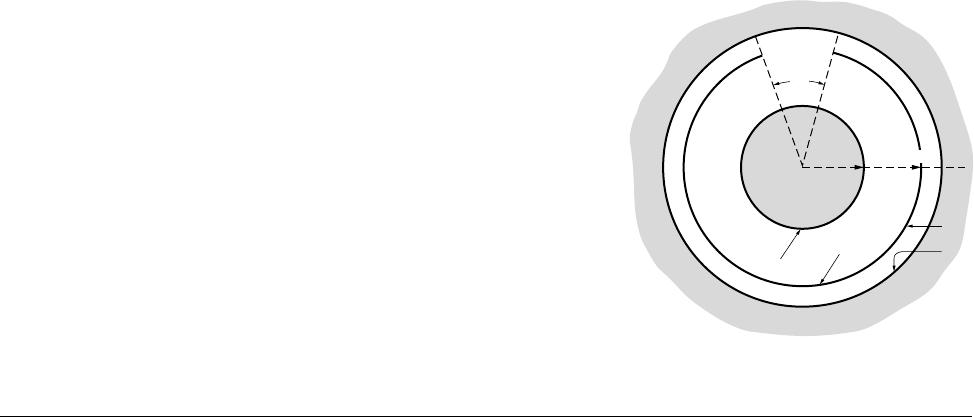5.38
2
A1
r2 = r3r4
r1
A2
A3
A4
θ
To reduce heat transfer between two infinite concentric cylin-
ders a third cylinder is placed between them as shown in the
figure. The center cylinder has an opening of half-angle θ. The
inner cylinder is black and at temperature T1=1000 K, while
the outer cylinder is at T4=300 K. Outer cylinder and both
sides of the shield are coated with a reflective material, such
that ǫc=ǫ2=ǫ3=ǫ4Determine the heat loss from the inner
cylinder as function of coating emittance ǫc, using
(b) the network analogy.
Solution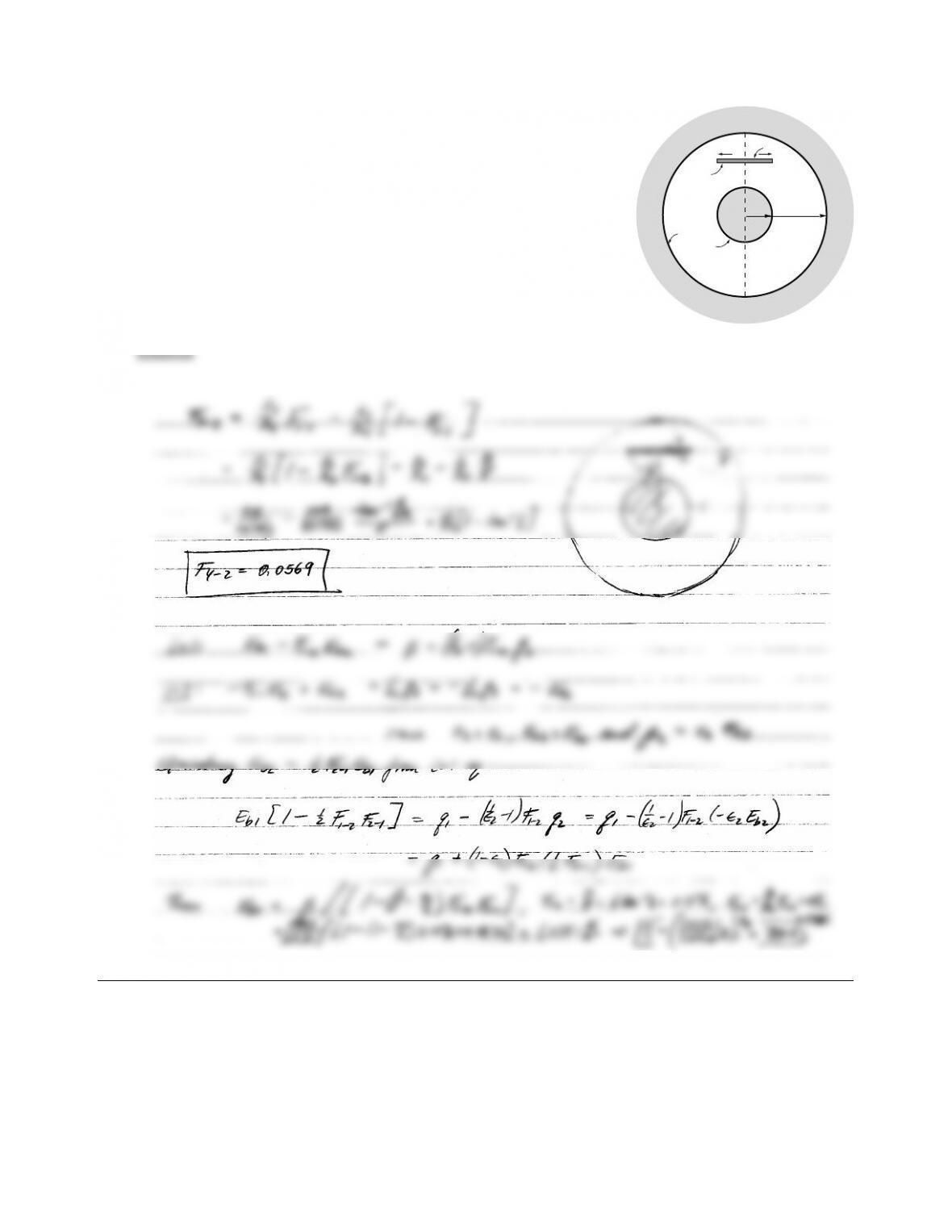CHAPTER 5 165
5.39
A1
A2
A4
R3R
R
2RA3
Consider the two long concentric cylinders as shown in the figure.
Between the two cylinders is a long, thin flat plate as also indicated.
The inner cylinder is black and generating heat on its inside in the
amount of Q
1=1 kW/m length of the cylinder, which must be removed
by radiation. The plate is gray and diuse with emittance ǫ2=ǫ3=0.5,
while the outer cylinder is black and cold (T4=0 K). Determine the
temperature of the inner cylinder, using
(b) the network analogy.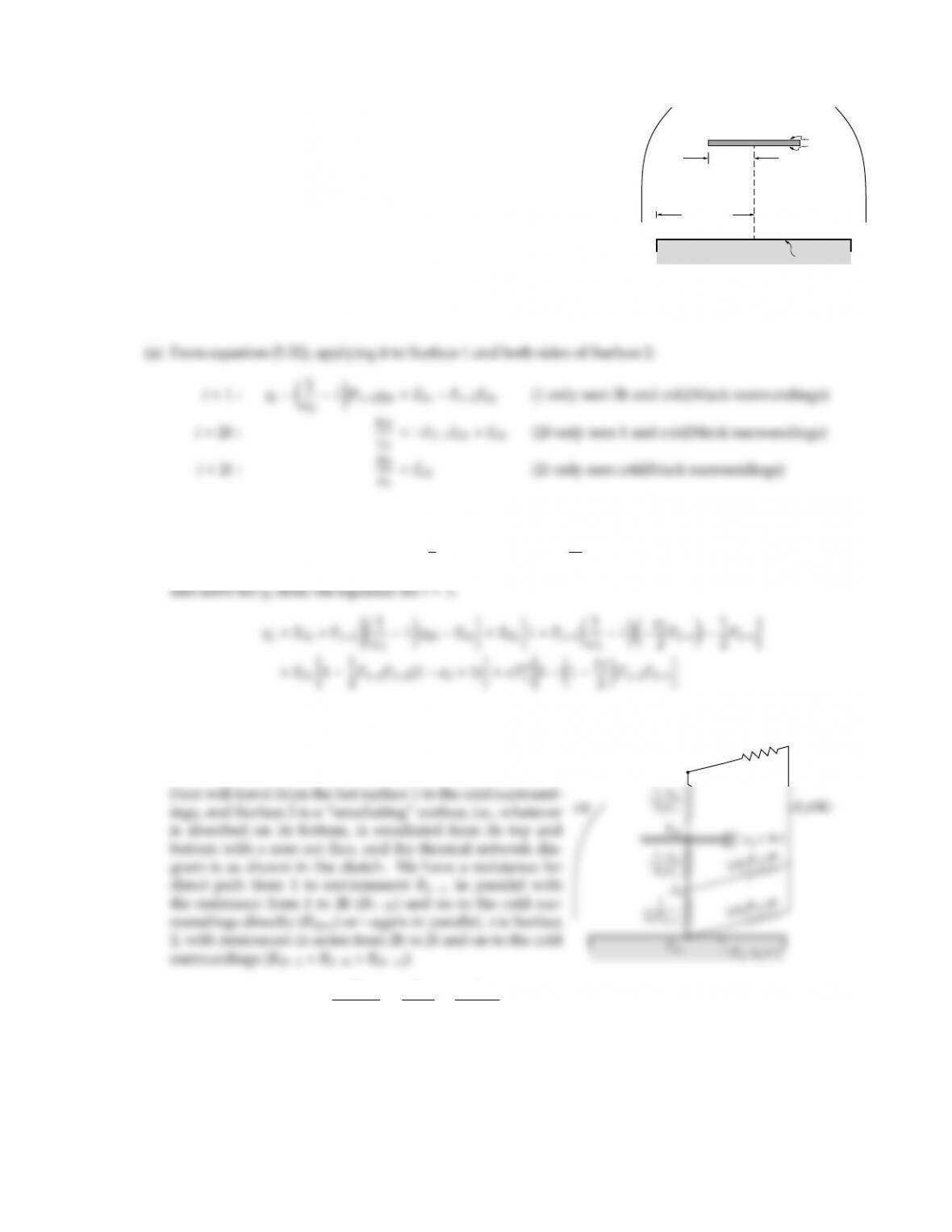5.40
R2 =?
h = ?
R1 = 0.1m
T1, 1 = 1
0 K
2 = 0.1
0 K
An isothermal black disk at T1=500Kis flush with the outer surface
of a spacecraft and is thus exposed to outer space. To minimize heat
loss from the disk a disk-shaped radiation shield is placed coaxially
and parallel to the disk as shown; the shield radius is R2(which may
be smaller or larger than R1), and its distance from the black disk is a
variable h. Determine an expression for the heat loss from the black
disk as a function of shield radius and distance, using
(b) the network analogy.
Solution
But q2b+q2t=0 and, therefore, after adding the equations for i=2band 2twe obtain
Eb2=1
2F21Eb1;q2b=ǫ2
2F21Eb1,
(b) Thermal resistance analogy:
1/A2F2t–0K
J2t
1
R1−∞,tot
=1
R1−∞
+1
R12−∞
;R12−∞ =R12b+R2b−∞,tot;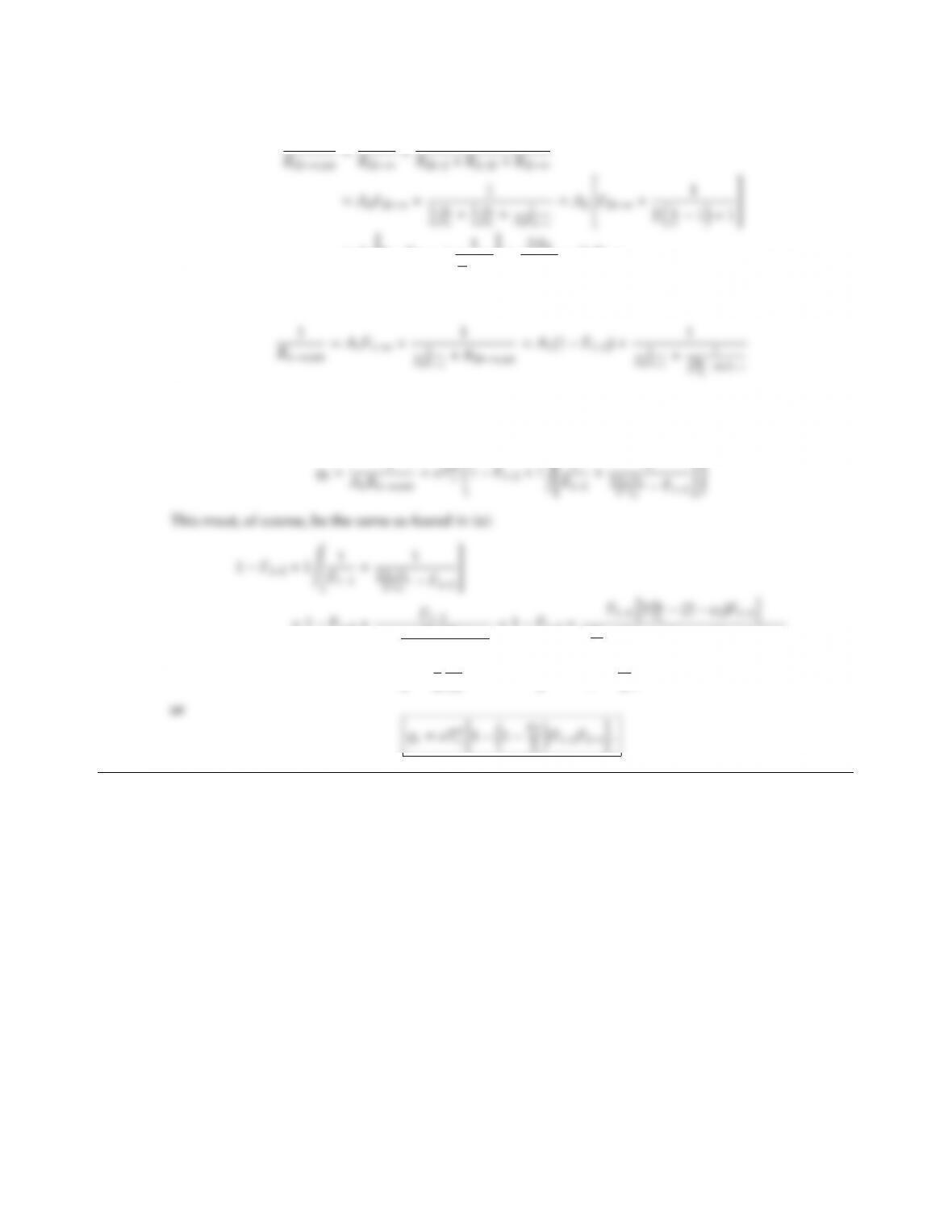CHAPTER 5 167
and
1
=A2
1F2b1+1
2
ǫ21
=2A2
2ǫ2A1F12;
and for the grand total resistance
The heat flux per unit area is then determined from
Eb1,=0
Eb
1
=1F12+F12
1+(2ǫ2)F12
2A2/A1(2ǫ2)F12
=1F12+F12h2A2
2A2
A1(2 ǫ2)F12+(2 ǫ2)F12
=1F12+F1211
2
A1
A2
(2 ǫ2)F12=11ǫ2
2F12F21

## Trusted by Thousands ofStudents

Here are what students say about us.